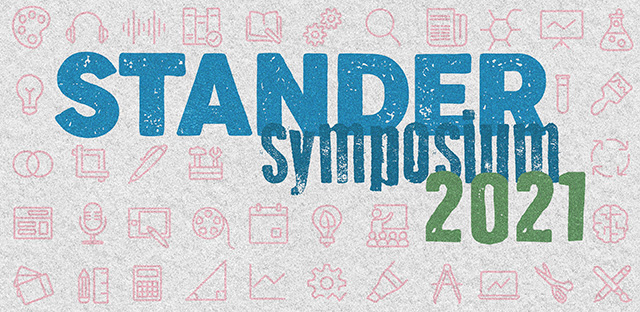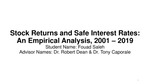## Stander Symposium Projects

#### Description

Stock Returns and Safe Interest Rates: An Empirical Analysis, 2001 – 2019Modern Finance Theory postulates that the intrinsic value of a firm's publicly traded common stock is equal to the discounted value of future cash flows or dividends. Holding cash flows constant, the intrinsic value depends on the interest rate at which the cash flows are discounted. A rise in the discount rate lowers the intrinsic value while a decrease in the discount rate increases the intrinsic value.In this study, I looked at the empirical relationship between intermediate and long-term treasury rates and the stock market as measured by the S&P 500 index. The period of analysis is 2001 – 2019 with a special focus on the empirical relationship pre and post 2008 recession. I use univariate regression analysis with the S&P 500 Index, or SPY, (in logs) as the Y variable and the intermediate (Treasury Notes) and Long-term (Treasury Bonds), also in logs, as the x variables. The hypothesis tested:1.The regression slope coefficients are statistically significant at the 95% confidence levels2.There is a recession effect i.e., the pre and post 2008 recession, slope coefficients are significantly different3.Since the long-term trend in treasury rates is downward sloping, the slope coefficients are positive i.e., lowering the discount rate results in rising stock prices.

4-22-2021

#### Project Designation

Independent Research

Tony S. Caporale, Robert D. Dean

Economics and Finance

#### Keywords